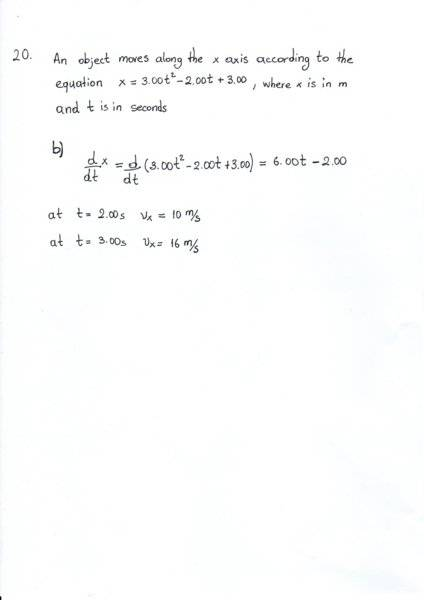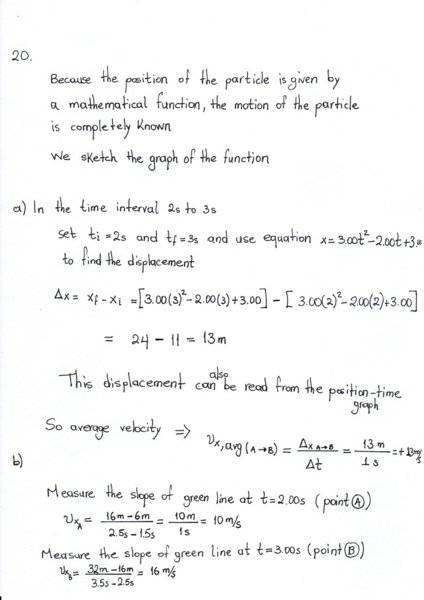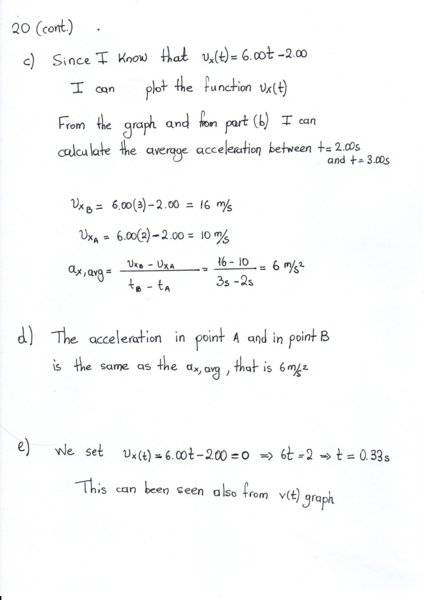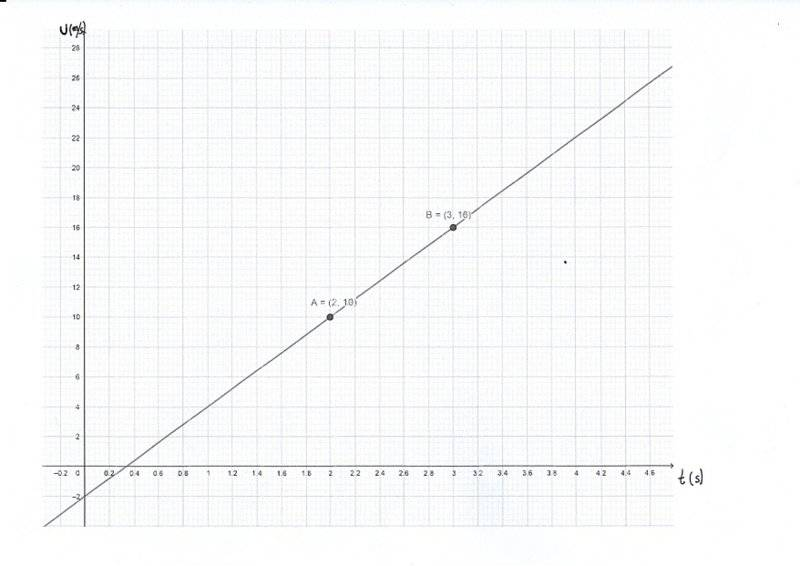# Calculate average speed/acceleration for the given function

## Homework Statement

Serway Physics Chapter 2

20. An object moves along the x axis according to the equation ##x=3.00t^2 - 2.00t+3.00##,
where x is in meters and t is in seconds. Determine (a) the average speed between
t=2.00s and t=3.00s, (b) the instantaneous speed at t = 2.00s and at t=3.00s, (c) the average acceleration
between t=2.00s and t=3.00s and (d) the instantaneous acceleration at t=2.00s and t=3.00s.
(e) At what time is the object at rest?

## The Attempt at a Solution[/B]
In part (a) I calculated average velocity but it asks for the average speed which is
given by ##v_{avg} = \frac {d} {Δt}## where d is distance travelled between 2 and 3 seconds, which is
13m. So average speed is the same as average velocity in this case.

Is the above correct?

DrClaude
Mentor
It all looks ok.